### What Is Inside Atom Class 9th Physics & Chemistry AP Board Solution

##### Class 9th Physics & Chemistry AP Board Solution

Question 1.

What are the three subatomic particles?

The particles present inside the atom are called as subatomic particles. The atoms are made up of three basic sub-atomic particles which are Proton, Electron and Neutron.

1. Electron: Negatively charged particle present outside the nucleus of an atom.

2. Proton: Protons are positively charged and are present inside the nucleus.

3. Neutron: Neutrons are neutrally charged particles, present within the nucleus of an atom.

The Figure below depicts the three subatomic particles present inside atom.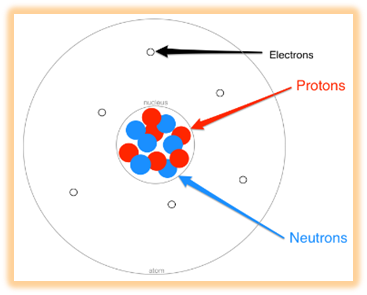Question 2.

Compare the characteristics of electrons, protons and neutrons.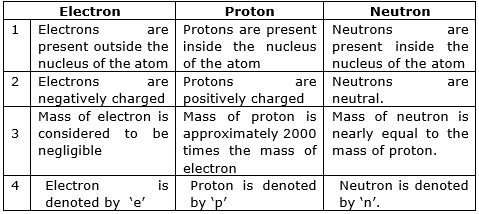Question 3.

What are the limitations of J.J. Thomson's model of the atom?

J.J Thomson considered atom as sphere of uniform distributed positive charge and electrons are embedded in it as shown in figure.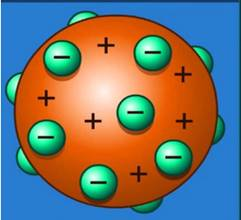The Limitation of this model are as follows.

1. J.J Thomson's model of the atom could not explain the results of Alpha particle scattering experiment carried out by Rutherford.

2. According to Thomson’s an atom consists of a positively charged sphere with electrons embedded in it. However, it was later found that the positively charged particles reside at the centre of the atom (nucleus), and the electrons revolve around the nucleus.

3. J.J Thomson's model of the atom was just based on imagination it did not have any experimental evidence in its support like the Rutherford's Model of the atom.

Question 4.

What were the three major observations Rutherford made in the gold foil experiment?

Ernest Rutherford was interested in knowing how the electrons are arranged within an atom. Therefore he designed an experiment for this. In this experiment, fast moving alpha (α)-particles were made to fall on a thin gold foil.

Three major observations that he made are as follows:

1. Most of the fast moving α-particles passed straight through the gold foil. The figure below shows the same.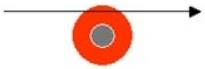2. Some of the α-particles were deflected by the foil by small angles. The figure below shows the same.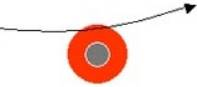3. Surprisingly one out of every 12,000 alpha particles appeared to rebound. The figure below shows the same.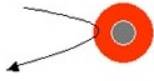Question 5.

Sketch Rutherford's atomic model. Why Rutherford's model of the atom is called the planetary model?

The Rutherford model described the atom as a tiny, dense, positively charged core called a nucleus, in which nearly all the mass is concentrated, around which the light, negative constituents called electrons circulate at some distance.

The model resemble much like our solar system in which sun in centre like nucleus and planets revolving around the sun in fixed orbits like electrons. Therefore it is called planetary model.

The Figure below is a sketch of Rutherford’s atomic model.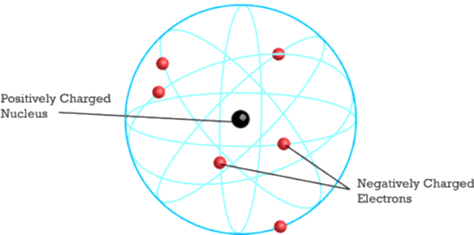Question 6.

Put tick (√) against correct choice and cross (×) against wrong choice:

In Rutherford's gold foil experiment, majority of alpha particles passed directly through the gold foil. This observation leads to which conclusion?

a) The positive region of the atom is very small.

b) The majority of the atom must consist of empty space.

c) The alpha particle makes a direct hit on the positive region.

d) The positive region of the atom is very dense.

a) The positive region of the atom is very small. √

b) The majority of the atom must consist of empty space. √

c) The alpha particle makes a direct hit on the positive region. ×

d) The positive region of the atom is very dense. ×

Explanation:

Option (a): since most of particles passed straight hence we can draw conclusion that positive region of the atom is very small.

Option (b): Most of the alpha particles passed straight through the atom without any deflection this lead to the conclusion that most of the atom consists of empty space. Just like if we throw a small stones (alpha particles) through the fence of considerably big gaps than most of the stones will pass through the gap.

Option (c): Since most of particles passed straight hence we can’t draw conclusion that alpha particle makes a direct hit on the positive region because if it had made direct hit it would have bounced back or changed its direction.

Option (d): since most of particles passed straight hence we can’t draw conclusion that positive region of the atom is very dense.

Question 7.

Put tick (√) against correct choice and cross (×) against wrong choice:

In Rutherford's gold foil experiment, occasionally the alpha particle veered from a straight-line path. This observation leads to which conclusion?

a) The positive region of the atom is very small.

b) The majority of the atom must consist of empty space.

c) The alpha particle makes a direct hit on the positive region.

d) The positive region of the atom is very dense

a) The positive region of the atom is very small. √

b) The majority of the atom must consist of empty space. X

c) The alpha particle makes a direct hit on the positive region. √

d) The positive region of the atom is very dense √

Explanation:

Option (a): since alpha particles veered from the path occasionally this lead to conclusion that the positive region of the atom is very small because if it would have been large the alpha particles would have deflected frequently.

Option (b): the conclusion that majority of the atom has empty space is derived from fact that most of particles passed straight without any deflection.

Option (c): the deviation of alpha particles from their straight path confirms that they must have been hit by a positive charged region (because alpha particles are also positively charged) and got repelled back.

Option (d): since the repulsion or deviation from the original path is strong it confirms that the positive region of the atom is very dense

Question 8.

Which one of the following is a correct electronic configuration of sodium?

(a) 2,8

(b) 8,2,1

(c) 2,1,8

(d) 2,8,1.

(d) 2, 8, 1.

Option (a): Atomic number of sodium is 11. Hence its atomic configuration also must have 11 electrons but 2,8 has only 10 electrons hence this option is incorrect.

Option (b): K shell can have maximum of 2 electrons but in this option K shell has 8 electrons .Hence it is incorrect.

Option (c): L shell can have maximum of 8 electrons and it needs to be filled first before filling M shell but in this option L shell has only 1 electron and M shell is filled before filling L shell. Hence this option is incorrect.

Option (d): since the atomic number of sodium is 11 and K, L, M shells can have maximum 2, 8, 18 electrons respectively. Therefore the correct electronic configuration of sodium is 2, 8, 1 where K shell has 2 electron, L shell has 8 electrons, M shell has 18 electrons. Therefore this option is correct.

Question 9.

Give the main postulates of Bohr's model of an atom.

To overcome the limitations of the Rutherford model of an atom in 1913 Neil’s Bohr suggested an atomic model which tells that electrons can be found only in certain energy levels around the nucleus. The main postulates of Bohr's model of an atom are as follows:

1. The electron revolves around the nucleus in certain discrete circular orbits of atom. These orbits or shells are called energy levels. The figure below shows energy levels of atom.

2. While revolving in these discrete orbits the electron do not radiate energy and this is the reason why electron do not fall in the nucleus.

3. The electron orbits or shells are represented by letters K,L,M,N etc or numbers n = 1,2,3 and so on this is shown in figure below.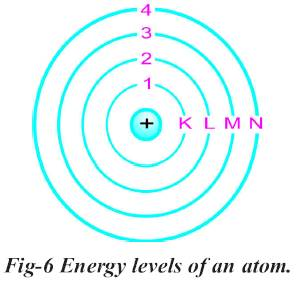4. An electron revolving in a particular orbit, in gaining a certain amount of energy, jumps to the next orbit and vice versa.

Question 10.

Compare all the proposed models of an atom given in this chapter.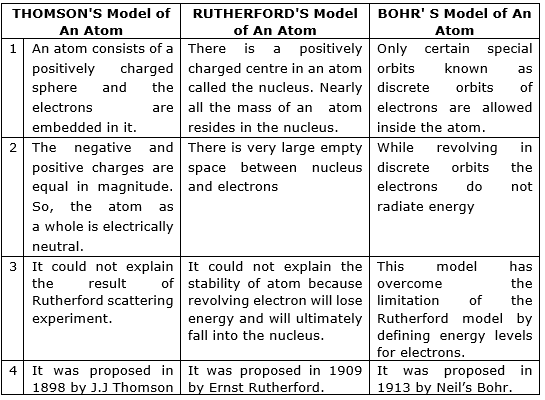The Picture below describes more clearly the difference between different proposed atomic models.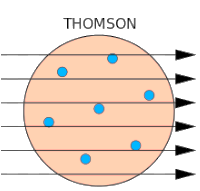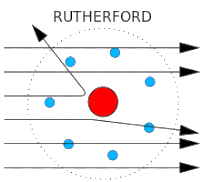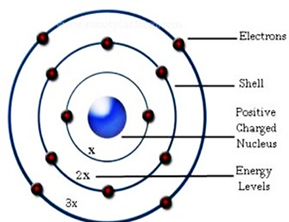NEILS BOHR MODEL

Question 11.

Define valency by taking examples of nitrogen and boron.

The valency may be defined as the combining capacity of the atoms of elements, or their tendency to form molecules with atoms of the same or different elements.

The electrons present in the outermost shell of an atom are known as the valence electrons. All atoms of various elements have tendency to have a fully-filled outermost shell with 8 electrons or octet. This is achieved through reaction with atoms of other elements, by sharing, gaining or losing electrons.

Example: NITROGEN: Atomic number of nitrogen is 7. Hence its electronic configuration is 2, 5. Hence to complete its octet it will take three electrons (5 + 3 = 8). Therefore its valency is 3 and negative (8 – 5).

BORON: Atomic number of boron is 5. Hence its electronic configuration is 2,3. Hence to complete its octet it will give three electrons Therefore its valency is 3 and positive.

Question 12.

State the valencies of the following elements : Magnesium and Sodium .

MAGNESIUM:Magnesium has an atomic number 12. Hence its electronic configuration is 2, 8, 2. Therefore it can lose two electrons to complete its octet (8 electrons in outermost shell). Hence its valency is 2

SODIUM: Sodium has an atomic number 11. Hence its electronic configuration is 2, 8, 1. Therefore it can lose one electrons to complete its octet (8 electrons in outermost shell). Hence its valency is 1.

Question 13.

If Z = 5, what would be the valency of the element?

Z denoted the atomic number of the element.

Therefore the element is Boron because atomic number of boron is 5.

Number of electrons Present in atom: 5;

Electronic configuration of atom: 2, 3.

Valency: since Boron has 3 electrons in its outer most shell .Hence it can give the three electrons in order to complete its octet. Therefore the valency of element is 3.

Question 14.

Write the atomic number and the symbol of an element which has mass number 32 and the number of neutrons 16 in the nucleus.

The relationship between Mass number, atomic number and number of neutrons is given by.

Number of neutrons (n) = Mass number (A) - Atomic number (Z)

We are given n = 16; A = 32

⇒ 16 = 32 – Z

⇒ Z = 32 – 16

⇒ Z = 16.

Hence the atomic number of element is 16.

Sulphur(S) is the element having atomic number 16.

Therefore symbol of the element is S.

Question 15.

Cl- has completely filled K&L shells. Explain.

Atomic number of chlorine ( Cl ) = 17.

Number of electrons in (Cl) = 17.

Electronic configuration of Cl = 2, 8, 7.

Cl- means negatively charged chlorine ion. It has gain one unit of negative charge by taking one electron.

∴ Number of electrons in (Cl-) = 18;

Electronic configuration of Cl- = 2, 8, 8.

We know that maximum numbers of electrons that can be filled in K and L shells are 8. And Cl- has 8 electrons in each K and L shells. Therefore the K and L shells of the Cl- are completely filled.

Question 16.

What is the main difference between isotopes of the same element?

DEFINITION: Two atoms that contain the same number of protons but different numbers of neutrons are isotopes of the same element. For example Deuterium and Tritium are isotopes of Hydrogen. Carbon-12, Carbon-13 and Carbon-14 are isotopes of carbon.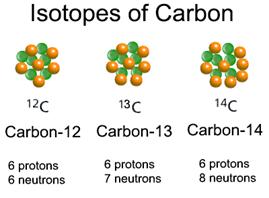DIFFERENCE: 1. Isotopes of the same element have different structure. Like Graphite, Diamond and Buckminster fullerene are isotopes of carbon but the difference is in their structure.

Fullerene is the network like structure similar to design in football. The figure below depicts the structure of fullerene.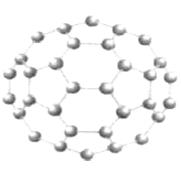In Diamond carbon is arranged in tetrahedral manner. The figure below depicts the structure of diamond.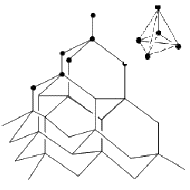In graphite carbon is layered structure so easy to break. The figure below depicts the structure of graphite.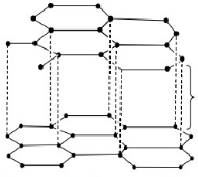2. The isotopes of the same element have different physical property but same chemical property.

Question 17.

For the following statements, write T for True and F for False.

a. J.J. Thomson proposed that the nucleus of an atom contains only nucleons.

b. A neutron is formed by an electron and a proton combining together. Therefore, it is neutral.

c. The mass of an electron is 1/2000 times that of proton.

(a) False J.J Thomson proposed that atom is a positively charged sphere with electrons embedded in it.

(b) False Neutron is one of the three independent sub atomic particles. It is the fundamental unit and is not form by combination of electron and proton.

(c) True It is a fact which is concluded from many experiments.

Question 18.

Fill in the missing information in the following table.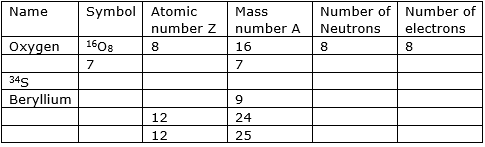Following concept will be used in filling the table

1. Number of Electrons = Atomic Number Z.

2. Number of neutrons (n) = Mass number (A) - Atomic number (Z).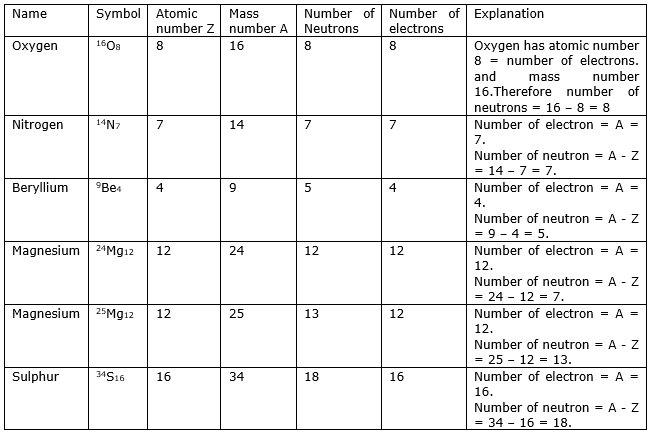Question 19.

How do you appreciate the efforts made by scientists to explain the structure of atom by developing various atomic models?

The existence of the atom has been proposed since the time of ancient Indian and Greek philosophers. But the atomic theory of matter was first proposed on scientific basis by John Dalton. After that many improvements were made in the proposed structure of atom and many new structures were also proposed some of them are as follows.

1. In 1898 J.J.Thomson discovered the electrons, which are in the atom. He stated the water melon structure of an atom.

2. Rutherford corrected the defects in Thomson’s model and introduced planetary atomic model. He observed the nucleus in the atom. He also explained the charge distribution.

3. Bohr corrected the defects on Rutherford’s model and explains the different energy levels in the atom.

4. All the discoveries made by the scientists are very helpful for further research and they are useful in understanding the molecules and atoms at microscopic level. We appreciate the role of scientists.

Question 20.

Geeta got a doubt, "Why atomic nucleus contains proton and neutrons inside it? Why can't electrons and neutrons?" Can you help to clarify her doubt? Explain.

Mass of electron is negligible compared to that of proton. Whereas the mass of proton is 2000 times of mass of electron.

Generally the heavy mass present in the nucleus. So the positive charged protons are present in the nucleus of an atom because of their heavy mass.

There exists force of repulsion between same charged particles. To reduce the repulsion force between protons in the nucleus, neutron exists in the nucleus. The electrons revolve around the nucleus in specified paths.

Question 21.

Collect information about various experiments conducted and theories proposed by scientists starting from John Dalton to Niels Bohr and prepare a story with a title "History of atom".

HISTORY OF ATOM

JOHN DALTON: from his experiments and observations, he suggested that atoms were very tiny particles and we cannot see it with our naked eye. Dalton believed that atoms were the fundamental building blocks of nature and could not be split. In chemical reactions.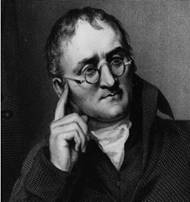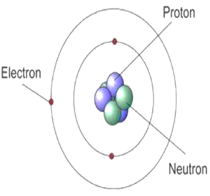J.J THOMSON: In 1898 J.J.Thomson discovered the electron. Thomson proposed a different model for the atom. He said that the tiny negatively charged electrons must be embedded in a cloud of positive charge like currants spread through a Christmas pudding.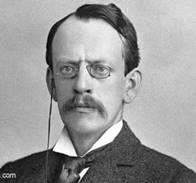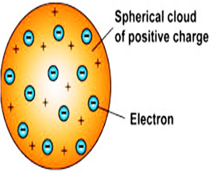ERNEST RUTHERFORD: In 1909, Ernest Rutherford performed the alpha particle scattering experiment. He said that Thomson's model could not be right. The positive charge must be concentrated in a tiny volume at the centre of the atom. On this model, the electrons orbited around the dense nucleus (centre of the atom).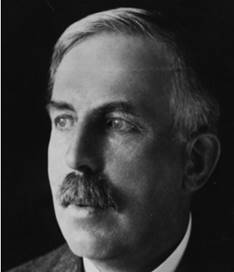ERNST RUTHERFORD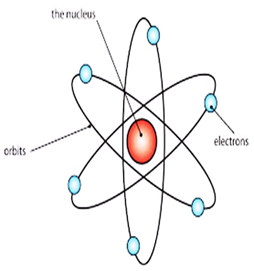ERNST RUTHERFORD MODEL

NIELS BOHR: The next important development came in 1914 when Danish physicist Niels Bohr revised the model again and overcome the drawback of instability of atom in Rutherford model. Bohr suggested that the electrons must be orbiting the nucleus in certain fixed energy levels (or shells). The energy must be given out when 'excited' electrons fall from a high energy level to a low one.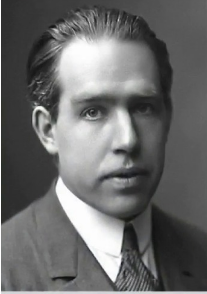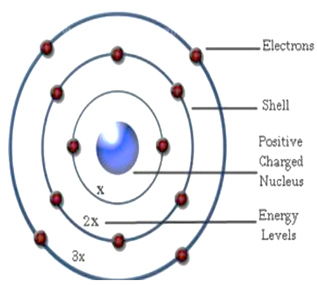## PDF FILE TO YOUR EMAIL IMMEDIATELY PURCHASE NOTES & PAPER SOLUTION. @ Rs. 50/- each (GST extra)

SUBJECTS

HINDI ENTIRE PAPER SOLUTION

MARATHI PAPER SOLUTION
SSC MATHS I PAPER SOLUTION
SSC MATHS II PAPER SOLUTION
SSC SCIENCE I PAPER SOLUTION
SSC SCIENCE II PAPER SOLUTION
SSC ENGLISH PAPER SOLUTION
SSC & HSC ENGLISH WRITING SKILL
HSC ACCOUNTS NOTES
HSC OCM NOTES
HSC ECONOMICS NOTES
HSC SECRETARIAL PRACTICE NOTES

2019 Board Paper Solution

HSC ENGLISH SET A 2019 21st February, 2019

HSC ENGLISH SET B 2019 21st February, 2019

HSC ENGLISH SET C 2019 21st February, 2019

HSC ENGLISH SET D 2019 21st February, 2019

SECRETARIAL PRACTICE (S.P) 2019 25th February, 2019

HSC XII PHYSICS 2019 25th February, 2019

CHEMISTRY XII HSC SOLUTION 27th, February, 2019

OCM PAPER SOLUTION 2019 27th, February, 2019

HSC MATHS PAPER SOLUTION COMMERCE, 2nd March, 2019

HSC MATHS PAPER SOLUTION SCIENCE 2nd, March, 2019

SSC ENGLISH STD 10 5TH MARCH, 2019.

HSC XII ACCOUNTS 2019 6th March, 2019

HSC XII BIOLOGY 2019 6TH March, 2019

HSC XII ECONOMICS 9Th March 2019

SSC Maths I March 2019 Solution 10th Standard11th, March, 2019

SSC MATHS II MARCH 2019 SOLUTION 10TH STD.13th March, 2019

SSC SCIENCE I MARCH 2019 SOLUTION 10TH STD. 15th March, 2019.

SSC SCIENCE II MARCH 2019 SOLUTION 10TH STD. 18th March, 2019.

SSC SOCIAL SCIENCE I MARCH 2019 SOLUTION20th March, 2019

SSC SOCIAL SCIENCE II MARCH 2019 SOLUTION, 22nd March, 2019

XII CBSE - BOARD - MARCH - 2019 ENGLISH - QP + SOLUTIONS, 2nd March, 2019

HSC Maharashtra Board Papers 2020

(Std 12th English Medium)

HSC ECONOMICS MARCH 2020

HSC OCM MARCH 2020

HSC ACCOUNTS MARCH 2020

HSC S.P. MARCH 2020

HSC ENGLISH MARCH 2020

HSC HINDI MARCH 2020

HSC MARATHI MARCH 2020

HSC MATHS MARCH 2020

SSC Maharashtra Board Papers 2020

(Std 10th English Medium)

English MARCH 2020

HindI MARCH 2020

Hindi (Composite) MARCH 2020

Marathi MARCH 2020

Mathematics (Paper 1) MARCH 2020

Mathematics (Paper 2) MARCH 2020

Sanskrit MARCH 2020

Important-formula

THANKS